Open in App
Not now

# Estimation of gaussian noise in noisy image using MATLAB

• Difficulty Level : Hard
• Last Updated : 10 Nov, 2021

Noise is something that is unwanted and makes it difficult to observe the details in the image. Image noise is a random variation of brightness or color information in images and is usually an aspect of electronic noise. It can be produced by the image sensor and circuitry of a scanner or digital camera.

An undesirable electrical fluctuation is also called “noise”.

A common type of noises:

• Rician noise: Rician noise is a type of artifact inherent to the acquisition process of the magnitude MRI image, making diagnosis difficult.
• Periodic noise: A common supply of periodic noise in an image is from electric or electromechanical interference at some point in the image capture process. A photograph affected by periodic noise will seem like a repeating sample has been introduced on top of the original image. In the frequency domain, this kind of noise may be visible as discrete spikes. Significant reduction of this noise may be finished via way of means of making use of notch filters in the frequency domain.
• Salt and pepper noise: This is a result of the random creation of pure white or black (high/low) pixels into the image. This is much less common in cutting-edge image sensors, even though can maximum usually be visible withinside the form of camera sensor faults (hot pixels which might be usually at most depth, or dead pixels which can be usually black). This kind of noise is also called impulse noise.
• Gaussian noise:  In this case, the random variant of the image signal around its expected value follows the Gaussian or normal distribution. This is the maximum usually used noise model in image processing and successfully describes most random noise encountered withinside the image-processing pipeline. This kind of noise is also called additive noise.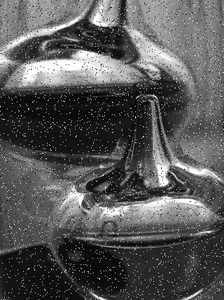Salt and pepper noise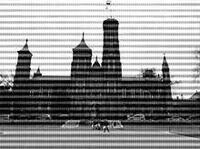Periodic noise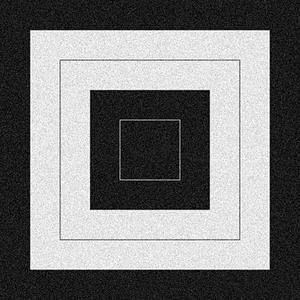Gaussian noise

Gaussian noise is statistical noise having a probability density function (PDF) equal to that of the normal distribution, which is also known as the Gaussian distribution. In other words, the values that the noise can take on are Gaussian-distributed. The mean of this noise is approx. zero. Sometimes it is called zero-mean Gaussian noise.

Example:

## Matlab

 `% MATLAB code for gaussian noise``% Reading the color image.``image=imread(``"img2.jfif"``);` `% converting into gray.``image=rgb2gray(image);` `% create the random gaussian noise of std=25``gaussian_noise=25*randn(size(image));` `% display the noise``imtool(gaussian_noise,[]);` `% display the gray image.``imtool(image,[]);`

Output: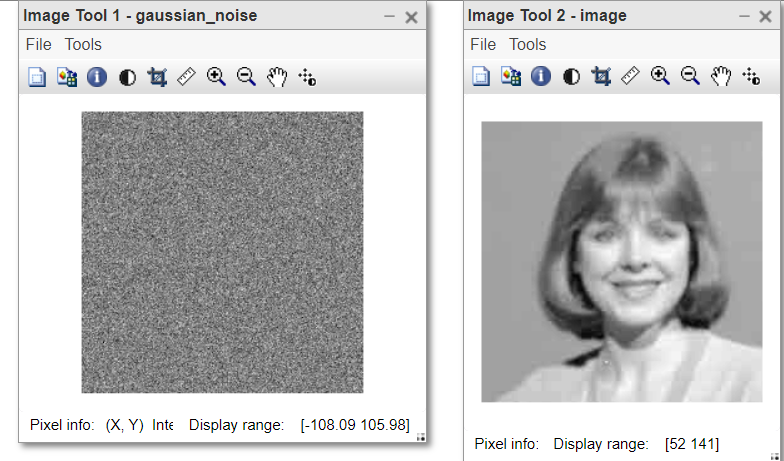Example:

## Matlab

 `% MATLAB code for demonstration of gaussian``% noisy image``% Reading the color image.``image=imread(``"k1.jfif"``);` `% converting into gray.``image=rgb2gray(image);` `% create the random gaussian noise of std=25``gaussian_noise=25*randn(size(image));` `% display the gray image.``imtool(image,[]);` `% add noise to the original image``noisy_image=double(image)+gaussian_noise;` `% display the noisy image``imtool(noisy_image,[]);`

Output: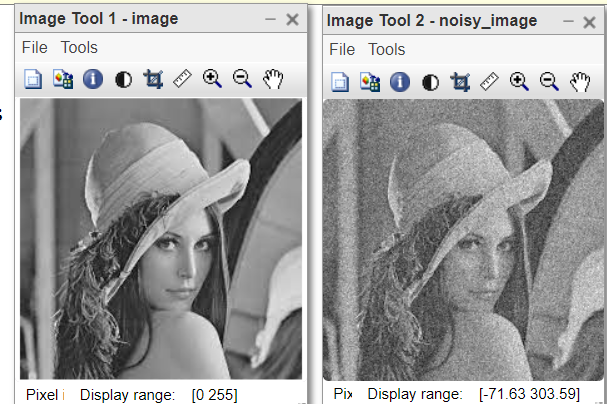Now we see how to estimate the level of Gaussian noise in the given image manually. For this firstly we generate the noisy image.

Example I:

## Matlab

 `% MATLAB code for estimate the level of Gaussian``% noise in the given image manually``% Reading the color image.``image=imread(``"cameraman.jpg"``);` `% converting into gray.``image=rgb2gray(image);` `% create the random gaussian noise of std=25``gaussian_noise=25*randn(size(image));` `% add noise to the original image``noisy_image=double(image)+gaussian_noise;` `% display the noisy image``imtool(noisy_image,[]);`

Output: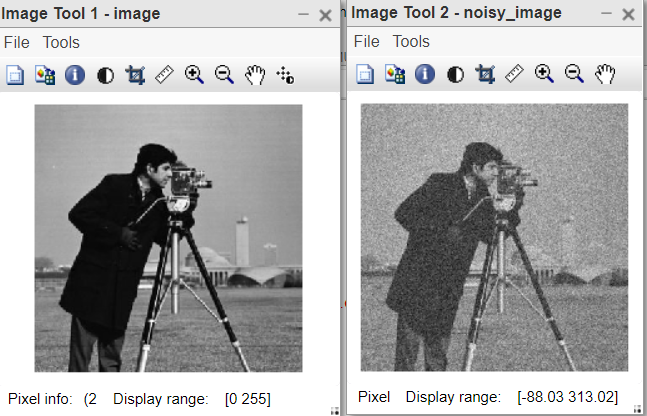The Gaussian noise is additive in nature. That means to create the noisy image, just add the noise in the original image.

Then, we crop the homogeneous part of the image and save that. Now find the standard deviation of that part, it will give us the estimation of gaussian noise in the noisy image.

Example II:

## Matlab

 `% MATLAB code for homogeneous part of the image``% and find the standard deviation of that part,``% it will give us the estimation of gaussian``% noise in the noisy image.``image=imread(``"cameraman.jpg"``);` `% create the random gaussian noise of std=25``gaussian_noise=25*randn(size(image));` `% display the gray image.``imtool(image,[]);` `% add noise to the original image``noisy_image=double(image)+gaussian_noise;` `% display the noisy image``imtool(noisy_image,[]);` `% crop the homogeneous part of the noisy image.``% display the cropped part.``imtool(cropped_image,[]);` `% standard deviation of the noise.``std(noise(:))` `% standard deviation of cropped homogeneous part.``std(cropped_image(:))`

Output: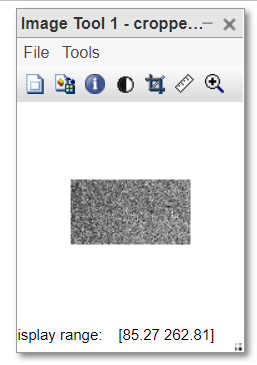Now verify the result. Match the standard deviation of the noise with the obtained result. If it is approximately equal means the homogeneous part cropped was correct otherwise choose the different homogeneous part. It requires expertise to find the perfect homogeneous part in the image.

That is why manual estimation is really time-consuming process. Next, we will see how to automate this.

### How to automate the estimation of gaussian noise in the image?

We will crop the homogeneous parts from the image and calculate their standard deviations. The homogeneous part of the image will always give the same standard deviation. So, in the list of many standard deviations, the most frequently occurring will belong to the homogeneous part or we can say noise.

Thus, the idea is to take the mode of the standard deviations obtained by the sliding window. This is the automatic approach.

Step 1: Create a sliding window. Slide it over the image and find the standard deviation of them.

Step 2: Find the mode.

Note: Choose the size of the sliding window carefully. It should be chosen with respect to the size of the original noisy image. Usually, [5, 5] window is the best choice.

Example:

## Matlab

 `% MATLAB code for automate the estimation``% of gaussian noise in the image``image=imread(``"cameraman.jpg"``);` `% create the random gaussian noise of std=25``gaussian_noise=25*randn(size(image));` `% add noise to the original image``noisy_image=double(image)+gaussian_noise;` `% std of noise.``std(noise(:)) ``%output=25.1444` `% list of std by using a sliding filter of [5 5].``list_of_std=uint8(colfilt(noisy_image, [5 5], ``'sliding'``, @std));` `% print the mode of this std list.``mode(list_of_std(:))`

Output:

Here, the standard deviation of the noisy image is estimated as 26.

My Personal Notes arrow_drop_up